# ski-in / ski-out - Page 22

Viewing mode
697 results
• Slopes access :
• ski-in (-50 m)
• Rooms :
• 5 rooms
• Total surface (sq.m) :
• 91 sq.m
•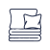•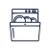•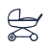•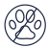• Slopes access :
• ski-in (-50 m)
• Rooms :
• 2 rooms
• 2 rooms + cabin(s)
• Total surface (sq.m) :
• 64 sq.m
•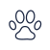•• Slopes access :
• ski-in (-50 m)
• Rooms :
• Studio
• Studio + cabin(s)
• Total surface (sq.m) :
• 34 sq.m
•••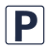••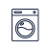• Slopes access :
• 50 -300m to the slopes
• Rooms :
• 2 rooms
• Total surface (sq.m) :
• 30 sq.m
•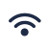••• Garage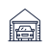•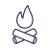•••• Slopes access :
• 50 -300m to the slopes
• Rooms :
• 2 rooms
• 2 rooms + cabin(s)
• Total surface (sq.m) :
• 38 sq.m
••••• Slopes access :
• ski-in (-50 m)
• Rooms :
• 2 rooms
• 2 rooms + cabin(s)
• Total surface (sq.m) :
• 38 sq.m
••••• Slopes access :
• ski-in (-50 m)
• Rooms :
• 3 rooms
• 3 rooms + cabin(s)
• Total surface (sq.m) :
• 75 sq.m
••••• Slopes access :
• ski-in (-50 m)
• Rooms :
• Studio
• Studio + cabin(s)
• Total surface (sq.m) :
• 32 sq.m
••••• Slopes access :
• 50 -300m to the slopes
• Rooms :
• 2 rooms
• 2 rooms + cabin(s)
• Total surface (sq.m) :
• 52 sq.m
••••• Slopes access :
• ski-in (-50 m)
• Rooms :
• Studio
• Studio + cabin(s)
• Total surface (sq.m) :
• 30 sq.m
••••• Slopes access :
• ski-in (-50 m)
• Rooms :
• Studio
• Studio + cabin(s)
• Total surface (sq.m) :
• 30 sq.m
••• Slopes access :
• ski-in (-50 m)
• Rooms :
• Studio
• Total surface (sq.m) :
• 32 sq.m
•••• Slopes access :
• ski-in (-50 m)
• Rooms :
• 2 rooms
• 2 rooms + cabin(s)
• Total surface (sq.m) :
• 40 sq.m
•••••• Slopes access :
• 50 -300m to the slopes
• Rooms :
• Studio
• Total surface (sq.m) :
• 33 sq.m
•••• Slopes access :
• ski-in (-50 m)
• Rooms :
• Studio
• Studio + cabin(s)
• Total surface (sq.m) :
• 27 sq.m
•••• Slopes access :
• 50 -300m to the slopes
• Rooms :
• Studio
• Total surface (sq.m) :
• 19 sq.m
••• Slopes access :
• ski-in (-50 m)
• Rooms :
• Studio
• Studio + cabin(s)
• Total surface (sq.m) :
• 27 sq.m
••••• Slopes access :
• ski-in (-50 m)
• Rooms :
• Studio
• Studio + cabin(s)
• Total surface (sq.m) :
• 33 sq.m
••••• Slopes access :
• ski-in (-50 m)
• Rooms :
• Studio
• Studio + cabin(s)
• Total surface (sq.m) :
• 27 sq.m
•••• Slopes access :
• ski-in (-50 m)
• Rooms :
• 2 rooms
• 2 rooms + cabin(s)
• Total surface (sq.m) :
• 63 sq.m
•••••